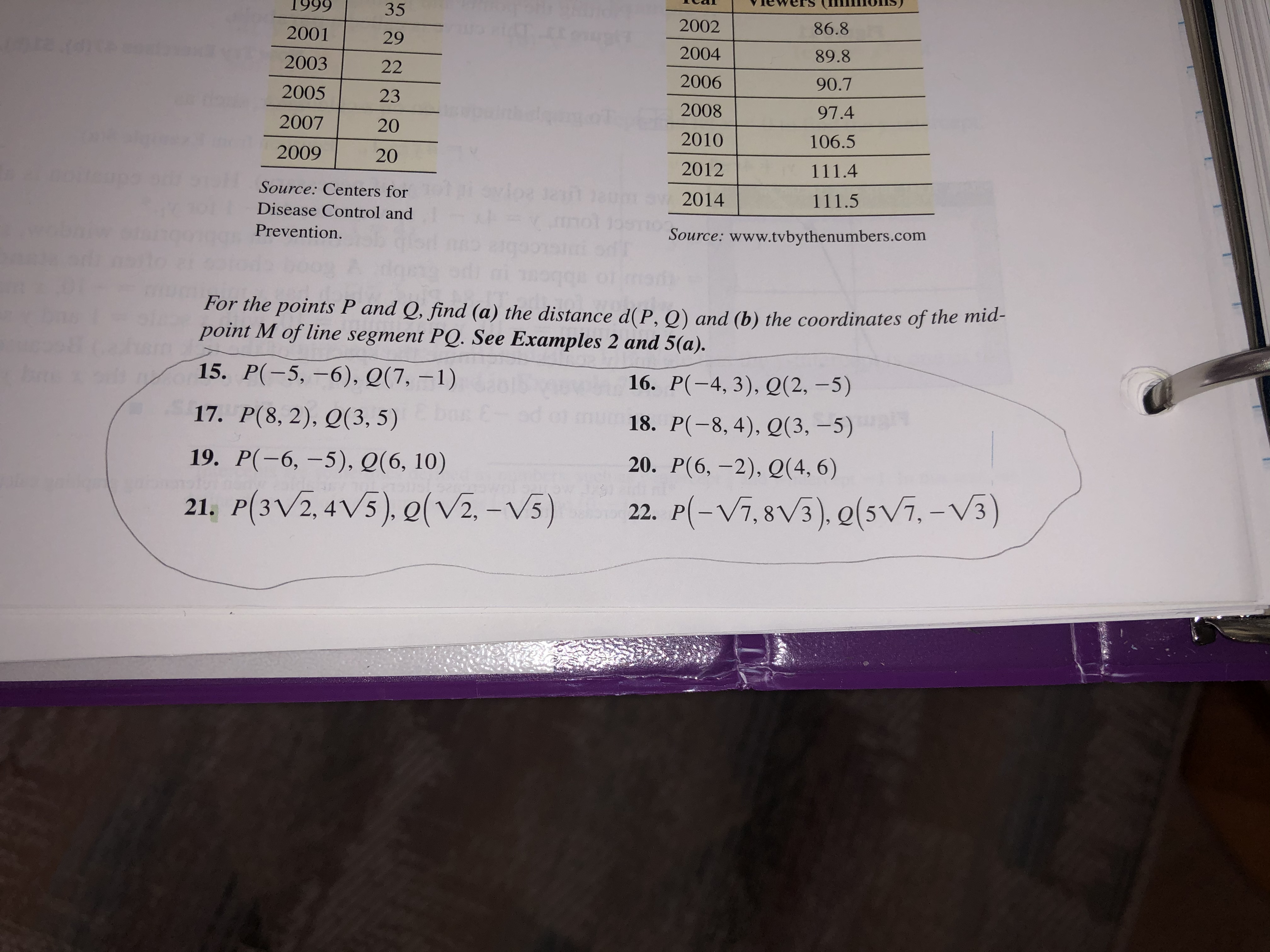# 199935200286.8200129200489.8200322200690.7200523200897.42007202010106.52009202012111.4Svloe 12 1atSource: Centers for111.52014Disease Control andSource: www.tvbythenumbers.comPrevention.For the points P and Q, find (a) the distance d(P, Q) and (b) the coordinates of the mid-point M of line segment PQ. See Examples 2 and 5(a).15. P(-5,-6), Q(7, -1)16. P(-4, 3), Q(2,-5)17. P(8, 2), Q(3, 5)18. P(-8, 4), Q(3, -5)20. P(6, -2), Q(4, 6)19. P(-6, -5), Q(6, 10)P-Vi.8V3), o(si.-V3)P(3V2, 4V5), (V2, -V5)22.21.

Question
1 viewshelp_outlineImage Transcriptionclose1999 35 2002 86.8 2001 29 2004 89.8 2003 22 2006 90.7 2005 23 2008 97.4 2007 20 2010 106.5 2009 20 2012 111.4 Svloe 12 1at Source: Centers for 111.5 2014 Disease Control and Source: www.tvbythenumbers.com Prevention. For the points P and Q, find (a) the distance d(P, Q) and (b) the coordinates of the mid- point M of line segment PQ. See Examples 2 and 5(a). 15. P(-5,-6), Q(7, -1) 16. P(-4, 3), Q(2,-5) 17. P(8, 2), Q(3, 5) 18. P(-8, 4), Q(3, -5) 20. P(6, -2), Q(4, 6) 19. P(-6, -5), Q(6, 10) P-Vi.8V3), o(si.-V3) P(3V2, 4V5), (V2, -V5) 22. 21. fullscreen
check_circle

Step 1

a)

The given points are P (–5, –6), and Q (7, –1).

Let (x1, y1) = (–5, –6) and (x2, y2) = (7, –1). Then x1 = –5, x2 = 7, y1 = –6 and y2 = –1.

Substitute the values x1 = –5, x2 = 7, y1 = –6 and y2 = –1 in the distance formula to find the distance as follows.

Step 2

Simplify the function as follows.

Step 3

(b)

The coordinates of the midpoint M of the line...

### Want to see the full answer?

See Solution

#### Want to see this answer and more?

Solutions are written by subject experts who are available 24/7. Questions are typically answered within 1 hour.*

See Solution
*Response times may vary by subject and question.
Tagged in

### Other